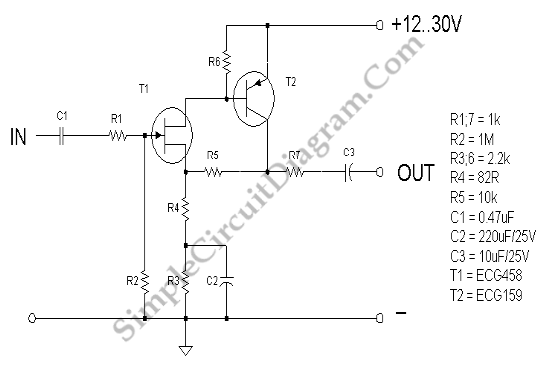# JFET Low Noise Preamp

Noise, in amplifier circuit, is generated intrinsically by each components inside the circuit. Resistor, capacitor, and semiconductor generate noises. By simplifying the design of a circuit, we can minimize the noise especially if we can push the number of components to the lowest count. Here is one example of such design.The gain of this low noise amplifier is determined by R5 and R4, follow this formula: gain=R5/(R4+R5).  This amplifier has non-inverting output, and high input impedance. Since the first stage of this amplifier uses JFET (ECG458)  which has high impedance, the input impedance is then primarily determined by R2, and 1 MΩ should be high enough for many application. R7 is employed to protect the circuit from overload (when the output is shorted to ground fro example). [Circuit’s schematic diagram source:lh-electric.4t.com]